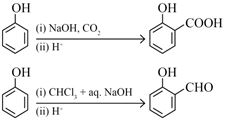﻿ Board Paper of Class 12 2022 Chemistry (Theory) Term-1 Code - (056/3/4) Set-4 Solutions | Aasoka

# Board Paper of Class 12 2022 Chemistry (Theory) Term-1 Code 056/3/4 Set-4

This section consists of 25 multiple choice questions with overall choice to attempt any 20 questions. In case more than desirable numbers of questions are attempted, ONLY first 20 will be considered for evaluation.

## Which one of the following pairs will form an ideal solution?

1. Chloroform and acetone
2. Ethanol and acetone
3. n-hexane and n-heptane
4. Phenol and aniline

Ans. (c)

Solution of n-hexane and n-heptane will form an ideal solution.

## Which of the following is known as amorphous solid?

1. Glass
2. Plastic
3. Rubber
4. All of the above

Ans. (d)

Glass, plastic and rubber are examples of amorphous solid.

## The structure of pyrosulphuric acid is

1.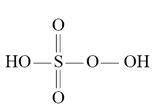2.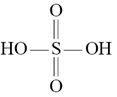3.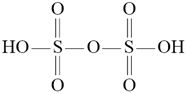4.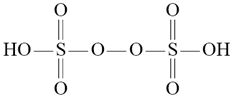Ans. (c)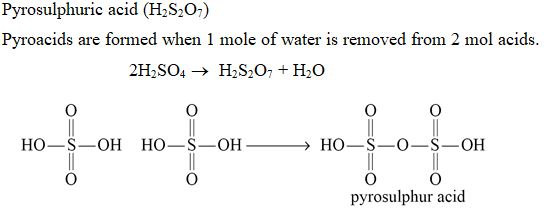## The C-O-H bond angle in alcohol is

1. slightly greater than 109°28'
2. slightly less than 109°28'.
3. slightly greater than 120°.
4. slightly less than 120°.

Ans. (b)

The C-O-H bond angle in alcohol is slightly less than 109°28’ due to repulsion between lone pair of oxygen.

## Consider the following reaction: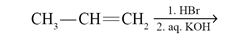The major end product is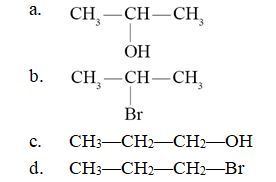Ans. (a)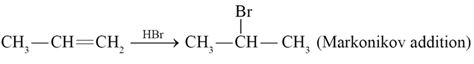## Nucleosides are composed of

1. a pentose sugar and phosphoric acid
2. a nitrogenous base and phosphoric acid
3. a nitrogenous base and a pentose sugar
4. a nitrogenous base, a pentose sugar and phosphoric acid

Ans. (c)

In a nucleoside, a base is attached at 1' position of pentose sugar.

## The oxidation state of -2 is most stable in:

1. O
2. S
3. Se
4. Te

Ans. (a)

O is most stable in -2 oxidation state due to its high electronegativity.

## Which of the following is not a characteristic of a crystalline solid?

1. A true solid
2. A regular arrangement of constituent particles
3. Sharp melting point
4. Isotropic in nature

Ans. (d)

Crystalline solids are anisotopic in nature. Some of their physical properties such as electrical resistance or refractive index show different in same crystal values when measured along different directions.

## Which of the following formula represents Raoult's law for a solution containing non-volatile solute?

1. psolute = p°solute . xsolute
2. p = KH.x
3. PTotal = psolvent
4. psolute = p°Solvent . xSolvent

Ans. (c)

PTotal = psolute + psolvent

Solute is non-volatile. So, psolute = 0.

Then, PTotal = psolvent

## An azeotropic solution of two liquids has a boiling point lower than either of the two when it

1. shows a positive deviation from Raoult's law.
2. shows a negative deviation from Raoult's law.
3. shows no deviation from Raoult's law.
4. is saturated.

Ans. (a)

In positive deviation from Raoult’s law, molecules of solution find it easier to escape than the constituent solutes. This will increase the vapour pressure and decrease the boiling point.

## Which of the following crystal will show metal excess defect due to extra cation?

1. AgCl
2. NaCl
3. FeO
4. ZnO

Ans. (d)

On heating ZnO loses oxygen and will show metal excess effect due to extra cation.

## Which of the following acids reacts with acetic anhydride to form a compound Aspirin?

1. Benzoic acid
2. Salicylic acid
3. Phthalic acid
4. Acetic acid

Ans. (b)

Salicylic acid reacts with acetic anhydride to form aspirin.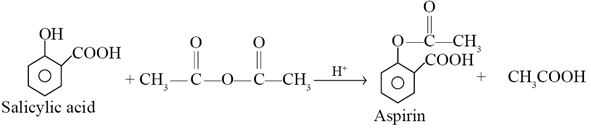## Which of the following statements is wrong?

1. Oxygen shows pπ - pπ bonding.
2. Sulphur shows little tendency of catenation.
3. Oxygen is diatomic whereas sulphur is polyatomic.
4. O-O bond is stronger than S-S bond.

Ans. (a)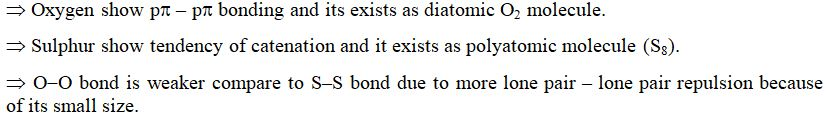## Amino acids which cannot be synthesized in the body and must be obtained through diet are known as

1. Acidic amino acids
2. Essential amino acids
3. Basic amino acids
4. Non-essential amino acids

Ans. (b)

Essential amino acids cannot be synthesized in body and must be obtained through diet.

## Which one of the following halides contains Csp2-X bond?

1. Allyl halide
2. Alkyl halide
3. Benzyl halide
4. Vinyl halide

Ans. (d)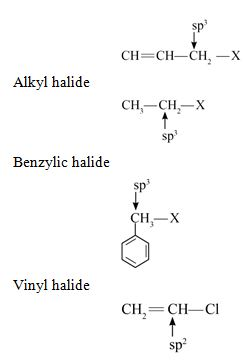## On mixing 20 mL of acetone with 30 mL of chloroform, the total volume of the solution is

1. $<$ 50 mL
2. = 50 mL
3. $>$ 50 mL
4. = 10 mL

Ans. (a)

Acetone and chloroform solution shows negative deviation from Raoult's law. In case of negative deviation, intermolecular attractive forces in solution are stronger than compare to components of solution. So, volume of solution decreases

Volume of solution $<$ Volume of solute + Volume of solvent

Volume of solution $<$ 20 + 30 ($<$ 50 ml)

## Consider the following compounds: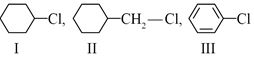The correct order of reactivity towards SN2 reaction:

1. I > III > II
2. II > III > I
3. II > I > III
4. III > I > II

Ans. (c)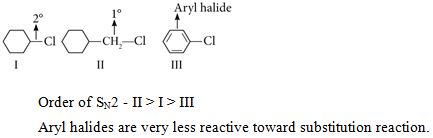## Which of the following forms strong p$\mathrm{\pi }$- p$\mathrm{\pi }$ bonding?

1. S8
2. Se8
3. Te8
4. O2

Ans. (d)

Due to small size of oxygen, only O2 will form strong p$\mathrm{\pi }$- p$\mathrm{\pi }$ bonding.

## F2 acts as a strong oxidising agent due to

1. low ${∆}_{\mathrm{bond}}$ H° and low ${∆}_{\mathrm{hyd}}$
2. low ${∆}_{\mathrm{bond}}$ H° and high ${∆}_{\mathrm{hyd}}$
3. high ${∆}_{\mathrm{bond}}$ H° and high ${∆}_{\mathrm{eg}}$
4. low ${∆}_{\mathrm{hyd}}$ H° and low ${∆}_{\mathrm{eg}}$

Ans. (b)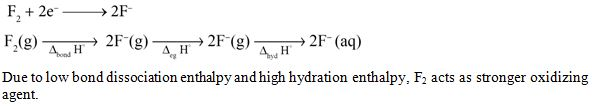## Which of the following sugar is known as dextrose?

1. Glucose
2. Fructose
3. Ribose
4. Sucrose

Ans. (a)

Glucose is known as dextrose.

## Cu reacts with dilute HNO3 to evolve which gas?

1. N2O
2. NO2
3. NO
4. N2

Ans. (c)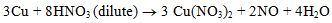## Which of the following is a network solid?

1. SO2
2. SiO2
3. CO2
4. H2O

Ans. (b)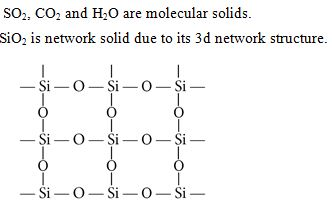## Major product formed in the following reaction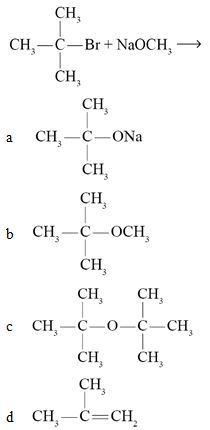Ans. (d)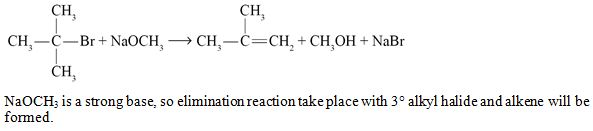## Chlorine reacts with cold and dilute NaOH to give

1. NaCl and NaClO3
2. NaCl and NaClO
3. NaCl and NaClO4
4. NaClO and NaClO3

Ans. (b)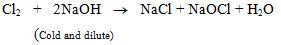## Elevation of boiling point is inversely proportional to

1. molal elevation constant (Kb)
2. molality (m)
3. molar mass of solute (M)
4. weight of solute (W)

Ans. (c)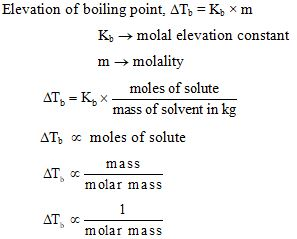This section consists of 24 multiple choice questions with overall choice to attempt any 20 questions. In case more than desirable numbers of questions are attempted, ONLY first 20 will be considered for evaluation.

## An unknown gas 'X' is dissolved in water at 2.5 bar pressure and has mole fraction 0.04 in solution. The mole fraction of 'X' gas when the pressure of gas is doubled at the same temperature is

1. 0.08
2. 0.04
3. 0.02
4. 0.92

Ans. (A)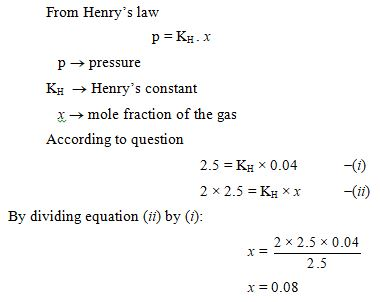## The base which is present in DNA but not in RNA, is

1. Cytosine
2. Guanine
4. Thymine

Ans. (d)

Bases present in RNA - adenine (A), guanine (G), cytosine (C), uracil (U)

## In the following reaction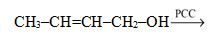the product formed is

1. CH3-CHO and CH3CH2OH
2. CH3-CH=CH-COOH
3. CH3-CH=CH-CHO
4. CH3-CH2-CH2-CHO

Ans. (c)

PCC is oxidising agent and it will oxidize primary alcohol to aldehyde.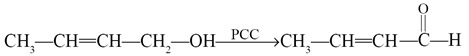## Enantiomers differ only in

1. boiling point
2. rotation of polarised light
3. melting point
4. solubility

Ans. (b)

Enantiomers have same physical properties but have different behavior toward plane polarised light.

## The number of lone pairs of electrons in XeF4 is

1. zero
2. one
3. two
4. three

Ans. (c)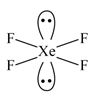## Sulphuric acid is used to prepare more volatile acids from their corresponding salts due to its

1. strong acidic nature
2. low volatility
3. strong affinity for water
4. ability to act as a dehydrating agent

Ans. (b)

Due to its low volatility sulphuric acid is used to prepare more volatile acids from their corresponding salts.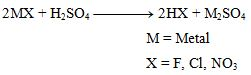## An element with density 6 g cm-3 forms a fcc lattice with edge length of 4 × 10-8 cm. The molar mass of the element is (NA = 6 × 1023 mol-1)

1. 57.6 g mol-1
2. 28.8 g mol-1
3. 82.6 g mol-1
4. 62 g mol-1

Ans. (a)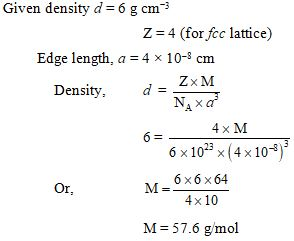##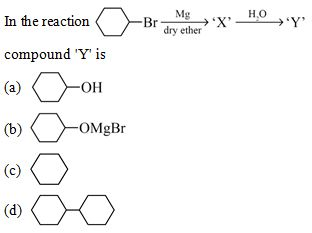Ans. (c)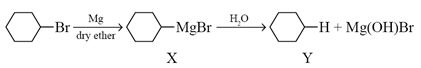## Which of the following is the weakest reducing agent in group 15?

1. NH3
2. PH3
3. AsH3
4. BiH3

Ans. (a)

NH3 is the weakest reducing agent due to its high bond dissociation enthalpy.

## The boiling point of a 0.2 m solution of a non-electrolyte in water is (Kb for water = 0.52 K kg mol-1)

1. 100 °C
2. 100.52 °C
3. 100.104 °C
4. 100.26 °C

Ans.(c)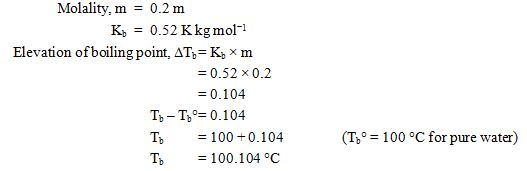## Nucleic acids are polymer of

1. amino acids
2. nucleosides
3. nucleotides
4. glucose

Ans. (c)

Nuclear acids are polymers of nucleotides

## Which of the following gas dimerises to become stable?

1. CO2 (g)
2. NO2 (g)
3. SO2 (g)
4. N2O (g)

Ans. (b)

NO2 (g) dimerises because it contain odd number of valence electrons.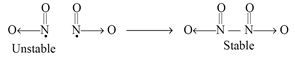## In the following diagram point, 'X' represents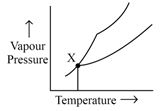1. Boiling point of solution
2. Freezing point of solvent
3. Boiling point of solvent
4. Freezing point of solution

Ans. (d)

Point X represent freezing point of solution.

## XeF6 on reaction with NaF gives

1. Na+[XeF7]-
2. [NaF2]- [XeF5]+
3. Na+[XeF6]-
4. [NaF2]+ [XeF5]-

Ans. (a)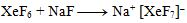## Glucose on reaction with Br2 water gives:

1. Saccharic acid
2. Hexanoic acid
3. Gluconic acid
4. Salicylic acid

Ans. (c)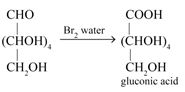## Which of the following is optically inactive?

1. (+) - Butan-2-ol
2. (-) - Butan-2-ol
3. $\left(±\right)$ - Butan-2-ol
4. (+) - 2-Bromobutane

Ans. (c)

$\left(±\right)$ - Buton-2-ol is optically inactive because it is a racemic mixture (50% R and 50% S).

## Which of the following is not a correct statement?

1. Halogens are strong oxidising agents.
2. Halogens are more reactive than interhalogens.
3. All halogens are coloured.
4. Halogens have maximum negative electron gain enthalpy.

Ans. (b)

Interhalogens are more reactive than halogens as X-X’ bond in interhalogens is weaker than X-X bond in halogens.

## Which of the following has highest boiling point?

1. C2H5-F
2. C2H5-Cl
3. C2H5-Br
4. C2H5-I

Ans. (d)

With increase in size, magnitude of van der Waals forces increases.

## Which of the following isomer of pentane (C5H12) will give three isomeric monochlorides on photochemical chlorination?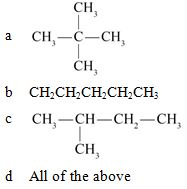Ans. (b)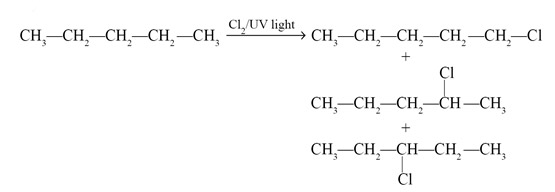Given below are the questions (45-49) labelled as Assertion (A) and Reason (R). Select the most appropriate answer from the options given below:

## Assertion (A): A raw mango placed in a saline solution loses water and shrivel into pickle.Reason (R): Through the process of reverse osmosis, raw mango shrivels into pickle.

1. Both A and R are true and R is the correct explanation of A.
2. Both A and R are true but R is not the correct explanation of A.
3. A is true but R is false.
4. A is false but R is true.

Ans. (c)

A raw mango placed in a saline solution loses water and shrivel into picked due to osmosis.

## Assertion (A): H2S is less acidic than H2Te. Reason (R): H-S bond has more ${∆}_{\mathrm{bond}}$ H° than H-Te bond..

1. Both A and R are true and R is the correct explanation of A.
2. Both A and R are true but R is not the correct explanation of A.
3. A is true but R is false.
4. A is false but R is true.

Ans. (a)

H2S is less acidic than H2Te due to more ${∆}_{\mathrm{bond}}$

## Assertion (A): Chlorobenzene is less reactive towards nucleophilic substitution reaction.Reason (R): Nitro group in chlorobenzene increases its reactivity towards nucleophilic substitution reaction.

1. Both A and R are true and R is the correct explanation of A.
2. Both A and R are true but R is not the correct explanation of A.
3. A is true but R is false.
4. A is false but R is true.

Ans. (b)

Chlorobenzene is less reactive toward nucleophilic substitution reaction due to partial double bond character in C–X bond. Electron withdrawing group such as nitro group increases the reactivity of haloarenes toward nucleophilic substitution reaction.

## Assertion (A): Due to Schottky defect, there is no effect on the density of a solid. Reason (R): Equal number of cations and anions are missing from their normal sites in Schottky defect.

1. Both A and R are true and R is the correct explanation of A.
2. Both A and R are true but R is not the correct explanation of A.
3. A is true but R is false.
4. A is false but R is true.

Ans. (d)

Due to Schottky defect, density of solid decreases.

## Assertion (A): Fluorine forms only one oxoacid HOF.Reason (R) : Fluorine atom is highly electronegative.

1. Both A and R are true and R is the correct explanation of A
2. Both A and R are true but R is not the correct explanation of A.
3. A is true but R is false.
4. A is false but R is true.

Ans. (a)

Fluorine forms only one oxoacid due to its high electronegativity and small size.

This section consists of 6 multiple choice questions with an overall choice to attempt any 5. In case more than desirable number of questions arc attempted, ONLY first 5 will be considered for evaluation.

## Match the following: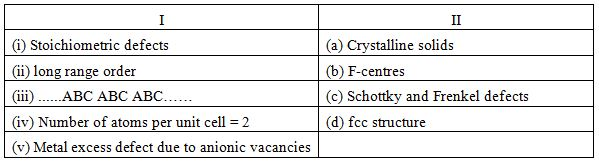Which of the following is the best matched options?

1. (i) - (d), (ii) - (a), (iii) - (b), (iv) - (c)
2. (i) - (c), (ii) - (a), (iii) - (d), (v) - (b)
3. (i) - (c), (ii) - (a), (iii) - (d), (iv) - (b)
4. (i) - (a), (ii) - (b), (v) - (c), (iv) - (d)

Ans. (b)

Stoichiometric defects - Schottky and Frenkel defects
Long range order - Crystalline solids
......ABC ABC ABC..... - fcc structure
Metal excess defect due to anionic vacancies - F-centres

## Which of the following analogies is correct?

1. XeF2 : linear :: XeF6 : square planar
2. moist SO2 : Reducing agent :: Cl2 : bleaching agent
3. N2 : Highly reactive gas :: F2 : inert at room temperature
4. NH3 : strong base :: HI : weak acid

Ans. (b)

moist SO2 : reducing agent :: Cl2 : bleaching agentb

## Complete the following analogy: Curdling of milk : A :: α-helix : B

1. A : Primary structure   B : Secondary structure
2. A : Denatured protein   B : Primary structure
3. A : Secondary structure   B : Denatured protein
4. A : Denatured protein   B : Secondary structure

Ans. (d)

Curdling of milk : Denatured protein :: α-helix : Secondary structure

Case: Read the passage given below and answer the following questions (53-55).

Alcohols and Phenols are acidic in nature. Electron withdrawing groups in phenol increase its acidic strength and electron donating groups decrease it. Alcohols undergo nucleophilic substitution with hydrogen halides to give alkyl halides. On oxidation primary alcohols yield aldehydes with mild oxidising agents and carboxylic acids with strong oxidising agents while secondary alcohols yield ketones. The presence of OH groups in phenols activates the ring towards electrophilic substitution. Various important products are obtained from phenol like salicylaldehyde, salicylic acid, picric acid etc.

## Which of the following alcohols is resistant to oxidation?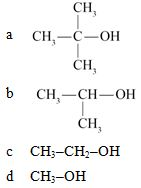Ans. (a)

Oxidation of tertiary alcohol does not take place.

## Which of the following group increases the acidic character of phenol?

1. CH3O -
2. CH3 -
3. NO2 -
4. All of these

Ans. (c)

Electron withdrawing group increases acidic strength and NO2 is a strong electron withdrawing group.

## Consider the following reaction: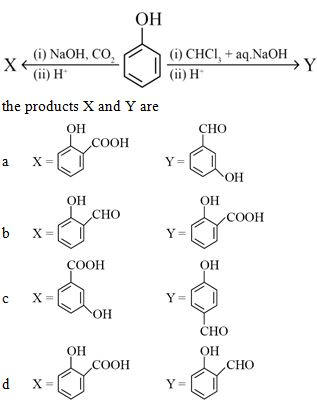Ans. (d)